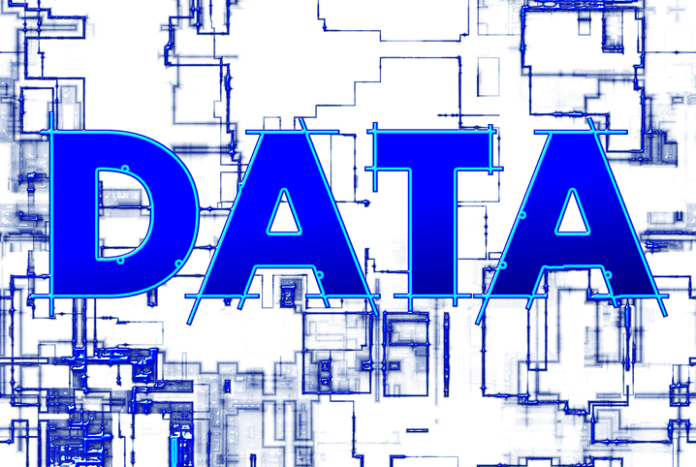# Types of Data in Statistics – A basic understanding for Machine Learning## Introduction

Machine Learning and data science thrives on data. It is very important to understand the nature of underlying data on top of which the machine learning model is required to be built. Given a dataset, one of the first thing you would normally do is try to understand the nature and variety of data present in the dataset. This usually becomes the first stepping stone of creating a powerful and robust machine learning model. Statistics is a distant cousin of machine learning which also deals with data. So if you can understand different types of data in Statistics field, you can apply the same knowledge for your machine learning problem.

Let us understand the different types of data you would normally encounter in Statistics.

### Two Main Types of Data

At the very high level you would generally encounter two types of data – numerical data or categorical.

1. Numerical – These types of data denotes numeric description of something. Examples of numerical data are age, weight, temperature, count of something etc. These types of data are also known as Quantitative data.
2. Categorical – These types of data describes the characteristics of something. Example of categorical data are gender of persons (Male or Female), names of places (India, America, England), color of car (Red, White). These types of data is also known as Qualitative data

Let us now take a deep dive in each of these types of data.

### Numerical Data

Numerical data can further be divided into following two types –

Discrete Numerical Data – These types of numeric data represent something which cant be divided into some meaningful parts. For example – number of children in a class. This can’t be fractional count like 40.5 , because 0.5 children is conceptually incorrect. Some other examples of discrete numerical data is – number of cars in parking area, number of states of country , count of animals in farm etc.

Continuous Numerical Data – These types of numeric data can be divided into further unit or can assume a more precision. These types of data is mostly a measurement of something. For example – an weight of a person can be 70.5 kg. Here fraction 0.5 kg is conceptually correct. This can also assume a more accurate precision like 70.56kg or 70.562kg.  Some other examples of continuous data is height, temperature, price etc. It is interesting to note that some types of data like Age, though can be divided into continuous representation is also used as discrete data in some problems.

### Categorical Data

Categorical Data can be further divided into –

Ordinal Data – These types of categorical data describes something and their ordering holds some significance. An example will make this clear. For example – Grades are categorical data which describes performance of a student. The possible values of Grades are given below.

Outstanding, Excellent, Average, Satisfactory, Failed

It is worth noting that the order of grades holds some significance, from left to right it represents the performance of students in decreasing scale.